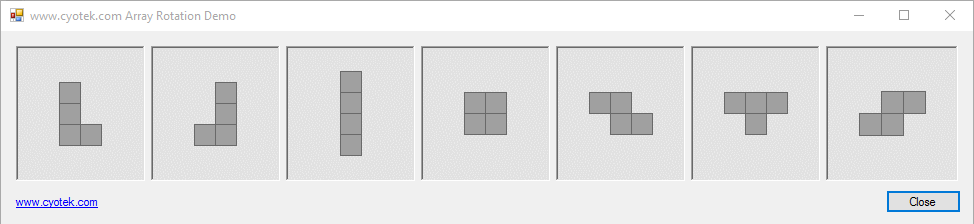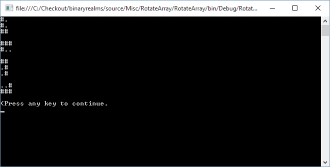## Rotating an array using C#

I've recently been working on a number of small test programs for the different sections which make up a game I'm planning on writing. One of these test systems involved a series of polyominoes which I needed to rotate. Internally, the data for these shapes are stored as a simple boolean array, which I access as though it were two dimensions.

One of the requirements was that the player needs to be able to rotate these shapes at 90° intervals, and so there were two ways I could have solved this

• Define pre-rotated versions of all shapes
• Rotate the shapes on the fly

Clearly, I went with option two otherwise there would be no need for this article! I choose not to go with the pre-rotated approach, as firstly I'm using a lot of shapes and creating up to 4 versions of each of these is not really worthwhile, and secondly I don't want to store them either, or have to care which orientation is currently in use.

This article describes how to rotate a 2D array in fixed 90° intervals, and also how to rotate 1D arrays that masquerade as 2D arrays.

Note: The code in this article will only work with rectangle arrays. I don't usually use jagged arrays, so this code has no special provisions to work with them.### Creating a simple sample

First up, we need an array to rotate. For the purposes of our demo, we'll use the following array - note that the width and the height of the array don't match.

``````bool[,] src;

src = new bool[2, 3];

src[0, 0] = true;
src[0, 1] = true;
src[0, 2] = true;
src[1, 2] = true;``````

We can visualize the contents of the array but dumping it in a friendly fashion to the console

``````private static void PrintArray(bool[,] src)
{
int width;
int height;

width = src.GetUpperBound(0);
height = src.GetUpperBound(1);

for (int row = 0; row < height + 1; row++)
{
for (int col = 0; col < width + 1; col++)
{
char c;

c = src[col, row] ? '#' : '.';

Console.Write(c);
}

Console.WriteLine();
}

Console.WriteLine();
}

PrintArray(src);``````

All of which provides the following stunning output

``````#.
#.
##
``````

### Rotating the array clockwiseThis function will rotate an array 90° clockwise

``````private static bool[,] RotateArrayClockwise(bool[,] src)
{
int width;
int height;
bool[,] dst;

width = src.GetUpperBound(0) + 1;
height = src.GetUpperBound(1) + 1;
dst = new bool[height, width];

for (int row = 0; row < height; row++)
{
for (int col = 0; col < width; col++)
{
int newRow;
int newCol;

newRow = col;
newCol = height - (row + 1);

dst[newCol, newRow] = src[col, row];
}
}

return dst;
}``````

How does it work? First we get the width and height of the array using the `GetUpperBound` method of the `Array` class. As arrays are zero based, we add `1` to each of these results, otherwise the new array will be too small to hold the data.

Next, we create a new array - with the width and height ready previously swapped, allowing us to correctly handle non-square arrays.

Finally, we loop through each row and each column. For each entry, we calculate the new row and column, then assign the value from the source array to the transposed location in the destination array

• To calculate the new row, we simply set the row to the existing column value
• To calculate the new column, we take the current row, add one to it, then subtract that value from the original array's height

If we now call `RotateArrayClockwise` using our source array, we'll get the following output

``````###
#..
``````

Perfect!

### Rotating the array anti-clockwise

Rotating the array anti-clockwise (or counter clockwise depending on your terminology) uses most of the same code as previous, but the calculation for the new row and column is slightly different

``````newRow = width - (col + 1);
newCol = row;``````
• To calculate the new row we take the current column, add one to it, then subtract that value from the original array's width
• The new column is the current row

Using our trusty source array, this is what we get

``````..#
###
``````

### Rotating 1D arrays

Rotating a 1D array follows the same principles outlined above, with the following differences

• As the array has only a single dimension, you cannot get the width and the height automatically - you must know these in advance
• When calculating the new index position using row-major order remember that as the width and the height have been swapped, the calculation will be something similar to `newIndex = newRow * height + newCol`

The following functions show how I rotate a 1D boolean array.

``````public Polyomino RotateAntiClockwise()
{
return this.Rotate(false);
}

public Polyomino RotateClockwise()
{
return this.Rotate(true);
}

private Polyomino Rotate(bool clockwise)
{
byte width;
byte height;
bool[] result;
bool[] matrix;

matrix = this.Matrix;
width = this.Width;
height = this.Height;
result = new bool[matrix.Length];

for (int row = 0; row < height; row++)
{
for (int col = 0; col < width; col++)
{
int index;

index = row * width + col;

if (matrix[index])
{
int newRow;
int newCol;
int newIndex;

if (clockwise)
{
newRow = col;
newCol = height - (row + 1);
}
else
{
newRow = width - (col + 1);
newCol = row;
}

newIndex = newRow * height + newCol;

result[newIndex] = true;
}
}
}

return new Polyomino(result, height, width);
}``````

Filename Description Version Release Date
RotateArray.zip
• sha256: 5ceae932d31aaf657e5c3dfc0d8908d52980bdfb7361bbc1473cfe5188ec4c0e

Sample project for the rotating an array using C# article.

1.0.0.0 24/12/2015